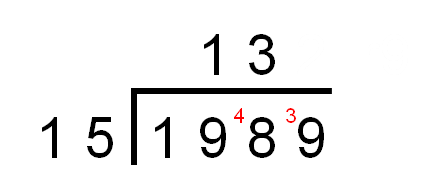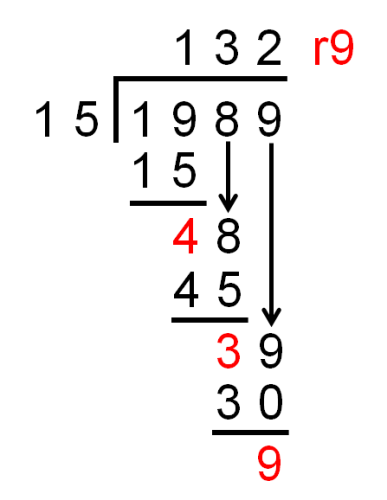• ## Accessibility

Open the accessibility toolbar to change fonts and contrast, access a dictionary, use a ruler and more

In this worksheet we will divide numbers with up to 4 digits by 2-digit numbers using long division.

There will be remainders which can be left as simple remainders.

Long Division is similar to Short Division, but more of the working is shown on paper.

Let's look at   1989 ÷ 15

Short Division

First we remind ourselves of how this would be worked out using Short Division.

We set out the calculation like this and then start dividing.First we divide 19 ÷ 15 = 1 rem 4.

(Remember that 1 × 15 = 15 and so the remainder is 19 - 15 = 4.)

The 4 goes next to the 8 to form 48.Then we divide 48 ÷ 15 = 3 rem 3.

(Remember that 3 × 15 = 45 and so the remainder is 48 - 45 = 3.)

The 3 goes next to the 9 to form 39.Lastly we divide 39 ÷ 15 = 2 rem 9.

(Remember that 2 × 15 = 30 and so the remainder is 39 - 30 = 9.)We have shown that    1989 ÷ 15 = 132 r9

Long Division

Now we look at the same division worked out using Long Division.

We start by setting out the calculation in the same way, like this, and then start dividing.First we divide 19 ÷ 15 = 1 rem 4.

(Remember that 1 × 15 = 15 and so the remainder is 19 - 15 = 4.)

In Long Division we actually set out the subtraction under the 19.In Short Division we put the 4 next to the 8 form 48.

In Long Division, we bring the 8 down next to the 4.Then we divide 48 ÷ 15 = 3 rem 3.

(Remember that 3 × 15 = 45 and so the remainder is 48 - 45 = 3.)

In Long Division, we bring the 9 down next to the 3.Lastly we divide 39 ÷ 15 = 2 rem 9.

(Remember that 2 × 15 = 30 and so the remainder is 39 - 30 = 9.)

The final remainder is 9.Compare this to short division.We have shown that    1989 ÷ 15 = 132 r9

Hint

With Long and Short Division, it helps to know the multiples of the number we are dividing by.

Here it helped to know the multiples of 15 are 15, 30, 45, 60, 75, 90 etc.

When dividing by 14, it helps to know the multiples of 14 are 14, 28, 42, 56, 70, 84 etc.

10 questions